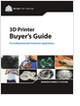MaterialsDesign CenterProcessesUnit ConversionFormulasMathematicsCalculatorsDiscussion ForumTrade PublicationsDirectory Service
Essentials of Manufacturing

Information, coverage of important developments and expert commentary in manufacturing.

CNC Machining Design Guide

3D Scanners

A white paper to assist in the evaluation of 3D scanning hardware solutions.

Selecting the Right 3D Printer

Discover how to choose the right 3D printer for your needs and the key performance attributes to consider.

more free publicationsGlossary » Units » Force » NewtonNewton (N) is a unit in the category of Force. It is also known as newtons. This unit is commonly used in the SI unit system. Newton (N) has a dimension of MLT-2 where M is mass, L is length, and T is time. This unit is the standard SI unit in this category.
Note that the seven base dimensions are M (Mass), L (Length), T (Time), Q (Temperature), N (Aamount of Substance), I (Electric Current), and J (Luminous Intensity).

Other units in the category of Force include A.u. of Force (a.u.), Crinal (crinal), Dyne (dyn), Gram Force (gf), Joule Per Centimeter (J/cm), Kilogram Force (kgf, kgp), Kilogram Meter Per Square Second (kg-m/s2), Kilopound Force (kip Force) (kipf), Ounce Force (av.) (ozf (av.)), Pound Force (lbf (av.), lbf), Pound Force Foot Per Inch (lbf-ft/in), Poundal (pdl), Slug Foot Per Square Second (slug-ft/s2), Slug Force, Square Foot Inch of Mercury (0°C) (ft2-inHg (0 °C)), Square Foot Inch of Mercury (15.56°C) (ft2-inHg (15.56 °C)), Ton Force (long), Ton Force (metric), and Ton Force (short).N/ARelated PagesGlossaryEssentials of Manufacturing

Information, coverage of important developments and expert commentary in manufacturing.CNC Machining Design Guide